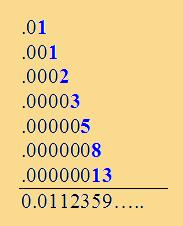# Fibonacci Numbers & the Fraction 1/89Most of us are familiar with the Fibonacci Sequence. This sequence is generated by starting with 2 numbers, 1 & 1 and generating the new terms by adding 2 previous terms. The sequence thus generated looks likeYou will notice that we started with two numbers 1 & 1. The new number is 1 + 1 = 2. The sequence thus becomes 1, 1, 2. The next number is 2 + 1 = 3. The sequence thus becomes 1, 1, 2, 3. The next number is 3 + 2 = 5. The sequence thus becomes 1, 1, 2, 3, 5. So on... So what is peculiar about 1/89 & this sequence? Here goes. Lets add up the numbers of this sequence in manner shown below:So, the sum looks like 0.0112359... Now, just fire up the calculator on your computer & find out what 1/89 turns out to be. Well, it turns out to be 0.0112359... Interesting, isnt it? If you are interested, feel free to go through my experiments (and results) with similar, Fibonacci-like sequences. Fibonacci numbers appear not only in mathematics, but are ubiquitous in the nature around us. Here's where you can know more about Fibonacci numbers in Nature To read more about Fibonacci numbers & their curious properties, here are some of the links you can visit http://pass.maths.org.uk/issue3/fibonacci/index.html http://en.wikipedia.org/wiki/Fibonacci_number
 Quick Comment : * Name Email ( Your email id is safe with us! ) * Comments * Type the number of characters in the word "HUMAN"